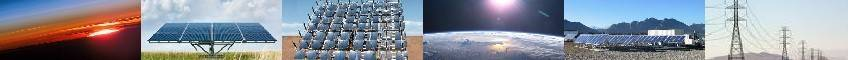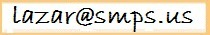# SOLAR BATTERY LIFE (RUNTIME) CALCULATOREstimation of a battery runtime (casually referred to as battery life) or selection of an energy storage system for a desired backup duration is a much more complicated task than one would think. We will analyze here some facts and common misconceptions. If you are not interested in technical analysis, you may just scroll down to the calculator.

Manufacturers normally advertise solar batteries by their so-called capacity. This quantity is expressed in ampere-hours (Ah) and is given for a specific discharge time at constant current and room temperature. By the way, our analysis applies not only to large storage systems, but also to small portable models, which are often listed by milli-amp-hours (mAh). Here are the main reasons why these ratings are not very helpful:
• Capacity is not constant-- it varies with the load and temperature;
• Batteries are practically never discharged at a constant current.

Let’s discuss these issues in more details.

Most often, the capacity is specified for 20-hour discharge (denoted by C/20). The consumers often do not realize that this quantity is not constant. For example, if a model is rated (say) for 100 Ah at C/20, this means it can supply 100/20=5 amps current for 20 hours. However, if you draw (say) 50 A, this battery may provide a backup only for a little more than one hour. The rule is, the faster the discharge the less actual amp-hours. Some lead acid batteries discharged in one hour provide only about 50% of their listed C/20 Ah ratings. Similarly, the runtime of uninterruptible power supplies (UPS) is usually given at half-load. At full load, they would typically run only 1/3 of their published time rather than ½.

## HOW TO CALCULATE BATTERY CAPACITY AT DIFFERENT "C" RATES?

A relationship between the amp-hours and discharge current is given by an empirical Peukert law. It states that: IK×t=constant, where K- is the Peukert coefficient, which is unique to a each make and model.
Some guides mistakenly say that the constant in the above equation is a capacity. It is not. We define the capacity as I×t. Only when I=1 amp, Peukert constant numerically equals to capacity. Since the manufacturers rarely specify it, how do we find battery life t at an arbitrary discharge current I? If tR is rated time to discharge at IR, then: IRKtR=IKt. Solving this for t yields:
t= IRKtR/IK
If we denote known capacity at a rated time tR by CR, then IR=CR/tR. Substituting this into above equation yields:
t=(CR/tR)KtR/ IK

This formula lets you calculate a battery life t at an arbitrary current I if you know its rated capacity CR at a rated time tR. There is still one unknown here- Peukert number K. Manufacturer datasheet often provides discharge graphs or Ah table for at different hour ratings. After some math, it can be shown that K can be calculated by using the following formula:
K = 1 + [Log (C2/C1)] / [Log (C1t2/C2t1)].
For example, Deka gel model 8G4D lists 169 Ah at 10 hr and 183 Ah at 20 hr. Then:
K=1 + [Log (183/169)] / [Log (169×20/183×10)]=1.13.
At 100 A load this model will run for (169/10)1.13×10/1001.13=1.34 hours. Of course, you don't want to run your battery flat, but during a blackout you may have no choice.
 Capacity (Ah) Rated time Peukert K Rated voltage Cutoff voltage Load (watts) Runtime, hrs
Note that capacity of lithium ion batteries is strongly dependent on their temperature. At a higher current, the temperature increases. This in turn increases the capacity, which partially compensates for power losses. As the result, for Li Ion K=1.01-1.04, so for simplicity you can assume K=1.

## HOW TO ACCOUNT FOR VARIABLE CURRENT?

So far we were considering discharge at a fixed current. In reality, there are three main reasons why a load always varies:
• Inverter produces AC output. Although its internal capacitors deliver a portion of AC current, the remaining portion is drawn from the input source.
• Motor-driven appliances (refrigerators, air conditioners) are periodically cycling on and off.
• Inverter regulates its output voltage. For a given load it supplies constant power. As battery is discharging its voltage gradually drops. As the result, inverter draws more current to meet the power demand.

So, what value of the current should we use in Peukert equation? The best thing you can do is use in the calculations average current based on average power. Let the loads in your home which you want to backup consume in average P watts, the battery initial terminal voltage is Vo and the cutoff voltage is Voff. Then use average current I=2P/(Vo+Voff) for your estimation. Note that P value should also take into an account inefficiency of your inverter.

The calculator to the right lets you estimate the run time of a fully charged battery. If you don’t know its cutoff voltage, assume it is 0.9 of nominal. Normally, 12V models are fully discharged at 10.5V. If you don’t know K, use the following typical values for different technologies: flooded lead-acid: 1.2-1.4; absorbed glass mat (AGM): 1.05-1.2; gel: 1.1-1.25. Note that these numbers may increase with the device age.

## WHAT BATTERY DO I NEED

Let's now solve a reverse task. Suppose you know your load wattage P and you picked a desired life time t. You want to find what storage capacity do you need. To determine this we need to solve the Peukert equation for C. Since most backup batteries are rated for 20-hr discharge, let tR=20. Also, let us assume Voff=0.9Vo, so that average voltage is 0.95Vo. Then the required 20-hr Ah rating is: C20=20×P/0.95Vo×(t/20)1/K.
Of course, to prolong the battery life cycle you don't want to discharge it more than 50-80%. So, ideally, when you are sizing your battery bank, you should double the result obtained from the above formula.

The information and suggestions here are provided AS IS for reference purpose only and do not constitute a professional or a legal advice. This is not an installation manual and is not intended to substitute or replace official codes and standards (see a complete legal disclaimer linked below).Disclaimer and Disclosure | Privacy